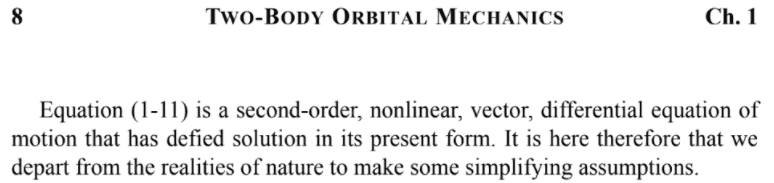# Two-body equation of motion resolution

Gold Member
Hi guys,

I have started studying differential equations on my own, sticking to my last high-school year's textbook, along with a few physics applications of ODEs.
Online I came across the n-body problem, which then took me to the basic two-body problem!

I'm here to ask you a few things about the two-body vector differential equation of motion:
$$\ddot{\textbf{r}}+\frac{\mu}{r^3}\textbf{r}=0$$

First: which type of DE is it? My textbook covers(approximately) linear DEs, explaining just a few resolution methods for first and second order homogeneous and non-homogeneous ones.
Is this non-linear, and so not solvable with "usual" and easier methods? If yes, why is it so?

Second: the textbook I found says: "though this equation looks easy, its complete solution is not".
Why?

Lastly, after the aforementioned consideration, it provides the steps to find a "partial solution that will tell us the size and shape of the orbit", which is a function ##r##(rel. position of the two bodies) of the form of a conic section: this "partial solution" thus tells us -only- the shape and size of the orbit, but doesn't provide us with a function of time for the movement.
How is that? What is a "partial solution", and what would be needed to find a "complete" one?

Online resources look all very confusing and over-complicated, and I'm having a really hard time deciphering those... hope you can help me, and my questions aren't too silly :)

Homework Helper
Hi,

First: which type of DE is it?
It took me a bit of searching to find your textbook. Next time, perhaps you can insert the link in your post ? Saves others time!

And on top of page 8 you missed the answer:The assumptions that follow and that lead to ##\ddot{\textbf{r}}+\frac{\mu}{r^3}\textbf{r}=0## don't change that.

Is this non-linear, and so not solvable with "usual" and easier methods? If yes, why is it so?

Second: the textbook I found says: "though this equation looks easy, its complete solution is not".
Why?
I could shrug this off with 'ours is not to reason why' or 'I really don't know ' . Why things are as they are is not physics. There are no tools in our toolkit to solve this analytically.

Suffice it to point to Kepler's equation ##M = E - e\sin E\ ## that remains after a whole lot of chewing.
One could argue that it's so difficult because the effective one-dimensional potential in which the motion takes place is such an unpleasant function of the radial distance ( ##-\displaystyle{a\over r} + \displaystyle{b\over r^2}\ ## ). Does that help you ?

The partial solution is 1-41 or 1-42 ( ##r = \displaystyle{p\over 1+e\cos\nu} \ ## ). The complete solution would be ##{\textbf{r}(t)}##, for which the book refers to Chapter 4, over a hundred pages further. You have your work cut out for you!

all very confusing and over-complicated, and I'm having a really hard time deciphering those... hope you can help me, and my questions aren't too silly :)
Your questions are not silly and I admire your courage and stamina to tackle something like this. But I think you are trying to bite off more than you can chew. I can't help in making it easier. The authors do a good job and I can't rewrite their book.

If your angle is physics stick with Mechanics_of_planar_particle_motion and Kepler's laws
And if it's differential equations, find an undergraduate calculus book you like and do the exercises.

##\ ##

Last edited:
•greg_rack and Ibix
Gold Member
Next time, perhaps you can insert the link in your post ? Saves others time!
Definitely will do! Haven't really thought about it before posting 😅

The assumptions that follow and that lead to ##\ddot{\textbf{r}}+\frac{\mu}{r^3}\textbf{r}=0## don't change that.
Great, and what does "defied solution" mean? I came across, I got the sense, but wasn't really able to get the precise meaning... I'm not English, and no translator gave me a satisfying translation for "defied solution"

One could argue that it's so difficult because the effective one-dimensional potential in which the motion takes place is such an unpleasant function of the radial distance ( ##-\displaystyle{a\over r} + \displaystyle{b\over r^2}\ ## ). Does that help you ?
That does definitely help, thanks a lot!

The partial solution is 1-41 or 1-42 ( ##r = \displaystyle{p\over 1+e\cos\nu} \ ## ).
Got it, but what is exactly meant by "partial solution"? Is it merely a way of solving the equation which gives the sought radius as a scalar, and not as ##{\textbf{r}(t)}##?

2022 Award
defied solution
It's a part of the verb "to defy". "It has resisted our attempts at finding a solution" is an expanded translation. Or "we can't solve it".

•greg_rack
Homework Helper
what is exactly meant by "partial solution"
Is is only a part of the solution, namely the radial distance as a function of an angle that varies in time.
So it gives the shape of the trajectory.
For a complete solution you would still need how that angle varies in time.

##\ ##

•greg_rack
VexCarido
Because the equation of the two body problem is what is known as a transcendental equation, and those often do not have a closed form solution. https://en.wikipedia.org/wiki/Transcendental_equation.

It is solvable though, but only using iterative calculation or geometry. If you just want to create some neat orbits then i suggest you use the Euler method instead of Keplers. Since the latter is probably a bit too difficult to start with.

Last edited:
•greg_rack
•